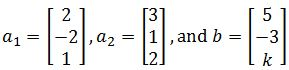# Linear combination and vector equations $R^n$

### Linear combination and vector equations $R^n$

#### Lessons

A matrix with one column is called a column vector. They can be added or subtracted with other column vectors as long as they have the same amount of rows.

Parallelogram Rule for Addition: if you have two vectors $u$ and $v$, then $u+v$ would be the fourth vertex of a parallelogram whose other vertices are $u,(0,0)$,and $v$

Here are the following algebraic properties of $\Bbb{R}^n$
1. $u+v=v+u$
2. $(u+v)+w=u+(v+w)$
3. $u+0=0+u=u$
4. $u+(-u)=-u+u=0$
5. $c(u+v)=cu+cv$
6. $(c+d)u=cu+du$
7. $c(du)=(cd)(u)$
8. $1u=u$

Given vectors $v_1,\cdots,v_p$ in $\Bbb{R}^n$ with scalars $c_1,\cdots,c_p$, the vector $x$ is defined by

$x=v_1 c_1+\cdots+v_p c_p$

Where $x$ is a linear combination of $v_1,\cdots,v_p$.

The linear combinations of $v_1,\cdots,v_p$ is the same as saying Span{$v_1,\cdots,v_p$}.
• Introduction
Vector Equations in $\Bbb{R}^n$ Overview:
a)
Vectors in $\Bbb{R}^2$
• Column vectors with 2 rows
• Adding, subtracting, and multiplying 2D vectors
• Graphing vectors in 2D

b)
Vectors in $\Bbb{R}^3$
• Column vectors with 3 rows
• Adding, subtracting, and multiplying 3D vectors
• Graphing vectors in 3D

c)
Vectors in $\Bbb{R}^n$
• Column vector with $n$ rows
• Algebraic properties

d)
Linear Combinations and Spans
• Vectors and weights
• Vector equations
• Finding a linear combination with row reduction

• 1.
Calculating Vectors in $\Bbb{R}^n$
Consider the two vectors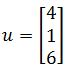, and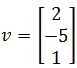. Compute:
a)
$u+2v$

b)
$2u-v$

c)
$5u+0v$

• 2.
Converting Systems Of Equations And Vector Equations
Write the given systems of equations as a vector equation.

$2x_1+x_2-5x_3=4$
$x_1+3x_2+2x_3=1$
$-4x_1-x_2-8x_3=-2$

• 3.
Write the given vector equation has a system of equations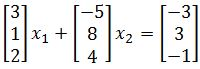• 4.
Linear Combinations with Known terms
Determine if $b$ is a linear combination of $a_1$, $a_2$ in part a. Determine if $b$ is a linear combination of $a_1$, $a_2$, and $a_3$ in part b and c.
a)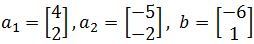b)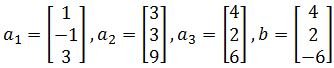c)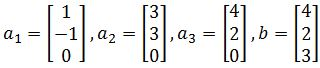• 5.
Linear Combinations with Unknown terms
For what value(s) of $k$ is $b$ in the plane spanned by $a_1$ and $a_2$ if: